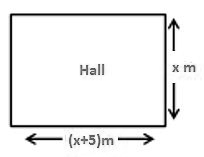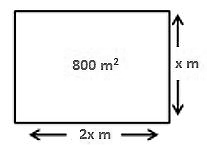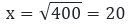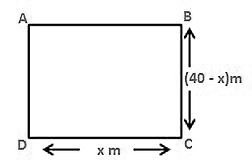×#### Thank you for registering.

One of our academic counsellors will contact you within 1 working day.

Click to Chat

1800-1023-196

+91-120-4616500

CART 0

• 0

MY CART (5)

Use Coupon: CART20 and get 20% off on all online Study Material

ITEM
DETAILS
MRP
DISCOUNT
FINAL PRICE
Total Price: Rs.

There are no items in this cart.
Continue Shopping• Complete JEE Main/Advanced Course and Test Series
• OFFERED PRICE: Rs. 15,900
• View Details

```Chapter 8: Quadratic Equations Exercise – 8.11

Question: 1The perimeter of the rectangular field is 82 m and its area is 400 m2. Find the breadth of the rectangle?

Solution:

Let the breadth of the rectangle be (x) m

Given,

Perimeter = 82 m

Area = 400 m2

Perimeter of a rectangle = 2(length + breadth)

80 = 2(length + x)

41 = (length + x)

Length = (41 - x)m

We know,

Area of the rectangle = length * breadth

400 = (41 - x)(x)

400 = 41x - x2

=  x2 - 41x +400 = 0

= x2 - 25x - 16x + 400 = 0

= x(x - 25) - 16(x - 25) = 0

= (x - 16)(x - 25) = 0

Either x - 16 = 0 therefore x = 16

Or, x - 25 = 0 therefore x = 25

Hence the breadth of the above mentioned rectangle is either 16 m or 25 m respectively.

Question: 2The length of the hall is 5 m more than its breadth. If the area of the floor of the hall is 84 m2, what is the length and breadth of the hall?

Solution:

Le the breadth of the rectangle be x m

Let the length of the hall is 5 m more than its breadth = (x + 5) m

Also given that,

Area of the hall is = 84 m2

The shape of the hall is rectangular

Area of the rectangular hall = length * breadth

84 = x(x + 5)

= x2 + 5x - 84 = 0

= x2 + 12x - 7x - 84 = 0

= x(x + 12) - 7(x + 12) = 0

= (x + 12)(x - 7) = 0

Either x + 12 = 0 therefore x = - 12

Or, x - 7 = 0 therefore x = 7

Since the value of x cannot be negative

So x = 7

= x + 5 = 12

The length and breadth of the rectangle is 7 and 12 respectively.

Question: 3

Two squares have sides x and (x + 4) cm. The sum of their area is 656 cm2. Find the sides of the square.

Solution:

Let S1 and S2 be the two square

Let x cm be the side square S1 and (x + 4) cm be the side of the square S2.

Area of the square S1 = x2 cm2

Area of the square S2 =(x + 4)2 cm2

According to the question,

Area of the square S1 + Area of the square S2 = 656 cm2

= x2 cm2 + (x + 4)2 cm2 = 656 cm2

= x2+ x2 + 16 + 8x - 656 = 0

= 2x2 + 16 + 8x - 656 = 0

= 2(x2 + 4x - 320) = 0

= x2 +4 x - 320 = 0

= x2 + 20x - 16x - 320 = 0

= x(x + 20) - 16(x + 20) = 0

= (x + 20)(x - 16) = 0

Either x + 20 = 0 therefore x = -20

Or, x - 16 = 0 therefore x = 16

Since the value of x cannot be negative so the value of x = 16

The side of the square S1= 16 cm

The side of the square S2 = 20 cm

Question: 4

The area of the right-angled triangle is 165 cm2. Determine the base and altitude if the latter exceeds the former by 7m.

Solution:

Let the altitude of the right angles triangle be denoted by x mGiven that the altitude exceeds the base by 7m = x - 7m

We know

Area of the triangle = 1/2 × base × altitude

= 165 = 1/2 × (x − 7) × x

= x(x - 7) = 330

= x2 - 7x - 330 = 0

= x2 - 22x + 15x - 330 = 0

= x(x - 22) + 15(x - 22) = 0

= (x - 22)(x + 15) = 0

Either x - 22 = 0 therefore x = 22

Or, x + 15 = 0 therefore x = -15

Since the value of x cannot be negative so the value of x = 22

= x - 7 = 15

The base and altitude of the right angled triangle are 15 cm and 22 cm respectively.

Question: 5

Is it possible to design a rectangular mango grove whose length is twice its breadth and area is 800 m2.find its length and breadth.

Solution:

Let the breadth of the rectangular mango grove be x mGiven that length of rectangle is twice of its breadth

Length = 2x

Area of the grove = 800 m2

We know,

Area of the rectangle = length * breadth

= 800 = x(2x)

= 2x2 - 800 = 0

= x2 - 400 = 0Breadth of the rectangular groove is 20 m

Length of the rectangular groove is 40 m

Yes, it is possible to design a rectangular groove whose length is twice of its breadth.

Question: 6

Is it possible to design a rectangular park of perimeter 80 m and area 400 m2?. If so find its length and breadth.

Solution:

In order to prove the given condition let us assume that the length of the rectangular park is denoted by x mGiven that,

Perimeter = 8 cm

Area = 400 cm2

Perimeter of the rectangle = 2(length + breadth)

We know,

Area of the rectangle = (length) (breadth)

= 400 = x(40 - x)

= 40x - x2 = 400

= x2 - 40x + 400 = 0

= x2 - 20x - 20x + 400 = 0

= x(x - 20) - 20(x - 20) = 0

= (x - 20)(x - 20) = 0

= (x - 20)2 = 0

= x - 20 = 0 therefore x =20

Length of the rectangular park is = 20 m

Breadth of the rectangular park = (40 - x) = 20 m

Yes, it is possible to design a rectangular Park of perimeter 80 m and area 400 m2

Question: 7

Sum of the area of the square is 640 m2.if the difference of their perimeter is 64 m, find the sides of the two squares.

Solution:

Let the two squares be S1 and S2 respectively.

Let he sides of the square S1 be x m and the sides of the square S2 be y m

Given that the difference of their perimeter is 64 m

We know that the

Perimeter of the square = 4(side)

Perimeter of the square S1 = 4x m

Perimeter of the square S2 = 4y m

Now, difference of their perimeter is 64 m

= 4x - 4y = 64

x - y = 16

x = y + 16

Also, given that the sum of their two areas

= area of the square 1 +area of the square 2

= 640 = x2 + y2

= 640 = (y + 16)2 + y2

= 2y2 + 32y + 256 - 640 = 0

= 2y2 + 32y - 384 = 0

= 2(y2 + 16y - 192) = 0

= y2 + 16y - 192 = 0

= y2 + 24y - 8y - 192 = 0

= y(y + 24) - 8(y + 24) = 0

= (y + 24)(y - 8) = 0

Either y + 24 = 0 therefore y = -24

Or, y - 8 = 0 therefore y = 8

Since the value of y cannot be negative so y = 8

Side of the square 1 = 8 m

Side of the square 2 = 8 + 16 = 24 m

The sides of the squares 1 and 2 are 8 and 24 respectively.
```### Course Features

• 728 Video Lectures
• Revision Notes
• Previous Year Papers
• Mind Map
• Study Planner
• NCERT Solutions
• Discussion Forum
• Test paper with Video Solution